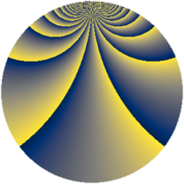# Properties

 Label 648.2.bdLevel $648$ Weight $2$ Character orbit 648.bd Rep. character $\chi_{648}(13,\cdot)$ Character field $\Q(\zeta_{54})$ Dimension $1908$ Newform subspaces $1$ Sturm bound $216$ Trace bound $0$

# Learn more

## Defining parameters

 Level: $$N$$ $$=$$ $$648 = 2^{3} \cdot 3^{4}$$ Weight: $$k$$ $$=$$ $$2$$ Character orbit: $$[\chi]$$ $$=$$ 648.bd (of order $$54$$ and degree $$18$$) Character conductor: $$\operatorname{cond}(\chi)$$ $$=$$ $$648$$ Character field: $$\Q(\zeta_{54})$$ Newform subspaces: $$1$$ Sturm bound: $$216$$ Trace bound: $$0$$

## Dimensions

The following table gives the dimensions of various subspaces of $$M_{2}(648, [\chi])$$.

Total New Old
Modular forms 1980 1980 0
Cusp forms 1908 1908 0
Eisenstein series 72 72 0

## Trace form

 $$1908q - 18q^{2} - 18q^{4} - 18q^{6} - 36q^{7} - 18q^{8} - 36q^{9} + O(q^{10})$$ $$1908q - 18q^{2} - 18q^{4} - 18q^{6} - 36q^{7} - 18q^{8} - 36q^{9} - 18q^{10} - 18q^{12} - 18q^{14} - 36q^{15} - 18q^{16} - 36q^{17} - 18q^{18} - 18q^{20} - 18q^{22} - 36q^{23} - 18q^{24} - 36q^{25} - 9q^{26} - 9q^{28} - 18q^{30} - 36q^{31} - 18q^{32} - 36q^{33} - 18q^{34} - 18q^{36} - 18q^{38} - 36q^{39} - 18q^{40} - 36q^{41} + 27q^{42} - 90q^{44} - 18q^{46} - 36q^{47} - 117q^{48} - 36q^{49} + 99q^{50} - 18q^{52} + 108q^{54} - 18q^{55} - 144q^{56} - 36q^{57} - 18q^{58} - 135q^{60} + 81q^{62} - 36q^{63} - 18q^{64} - 36q^{65} + 54q^{66} - 63q^{68} - 18q^{70} - 36q^{71} - 18q^{72} - 36q^{73} - 18q^{74} - 18q^{76} - 45q^{78} - 36q^{79} - 36q^{80} - 36q^{81} - 36q^{82} - 45q^{84} - 18q^{86} - 36q^{87} - 18q^{88} - 36q^{89} - 81q^{90} - 108q^{92} - 18q^{94} - 36q^{95} - 135q^{96} - 36q^{97} - 189q^{98} + O(q^{100})$$

## Decomposition of $$S_{2}^{\mathrm{new}}(648, [\chi])$$ into newform subspaces

Label Dim. $$A$$ Field CM Traces $q$-expansion
$$a_2$$ $$a_3$$ $$a_5$$ $$a_7$$
648.2.bd.a $$1908$$ $$5.174$$ None $$-18$$ $$0$$ $$0$$ $$-36$$